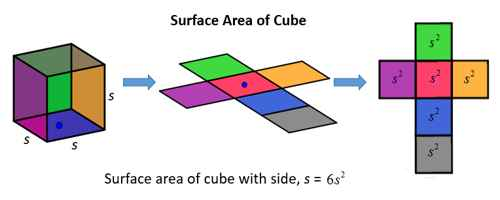Calculate the Surface Area of a Cube

To calculate the surface are of a cube, find the surface area of one side and multiply by 6. The surface area of any side is the length of a side squared.

Example: Surface area of a cube with a side of length 4 = 4*4*6 = 96

In words, the surface area of a cube is the area of the six squares that cover it. The area of one of them is a*a, or a2. Since these are all the same, you can multiply one of them by six, so the surface area of a cube is 6 times one of the sides squared.

Surface area = 6 a2

Where a, is the length of any edge of the cube.Example:

Find the surface area if the length of one side is 3 cm

Surface area = 6 × a2

Surface area = 6 × 32

Surface area = 6 × 3 × 3

Surface area = 54 cm2

Example 2: The surface area of a cube is 86.64 square meters. Determine the side length of the cube.

Solution: Because we already know the surface area, we can work backwards through the steps to determine the length of the side.

The side length was squared and then multiplied by 6. So we will divide by 6 to undo the multiplying. Then we will take the square root to undo the squaring.

86.64 / 6 = 14.44

= √14.44 = 3.8

Therefore, the sides of the cube are each 3.8 meters long.

To determine the surface area of a cube, calculate the area of one of the square sides, then multiply by 6 because there are 6 sides. This is the same as solving using the formula SA = 6s2. If you are given the surface area, you can determine the side length by working backwards. Take the surface area and work backwards by dividing by 6 and then take the square root.

Information Source: